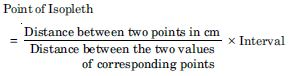# NCERT Solutions for Class 12 Geography Chapter 3 - Graphical Representation of Data

##### Question 1:

Which one of the following map shows the population distribution?

1. Choropleth maps
2. Isopleth maps
3. Dot maps
4. Square root map

(c) Dot maps

##### Question 2:

Which one of the following is best suited to represent the decadal growth of population?

1. Line graph
2. Bar diagram
3. Circle diagram
4. Flow diagram

(a) Flow diagram

##### Question 3:

Polygraph is constructed to represent:

1. Only one variable
2. Two variables only
3. More than two variables
4. None of the above

(d) None of the above

##### Question 4:

Which one the following maps is known as ‘Dynamic Map’?

1. Dot Map
2. Choropleth
3. Isopleth
4. Flow Map

(d) Flow Map

##### Question 5:

What is thematic map?

A thematic map is a map specifically designed to show a particular theme connected with a specific geographic area. A thematic map is a map that focuses on a specific theme or a subject area.

##### Question 6:

Differentiate between multiple bar diagram and compound bar diagram.

Bar diagram: The bar diagrams are drawn through columns of equal width. It is also called a column diagram.
Compound bar diagram: When different components are grouped in one set of variable or different variables of one component are put together, their representation is made by a compound bar diagram. In this method different variables are shown in the single bar with different rectangles.

##### Question 7:

What are the requirements to construct a dot map?

Requirements to construct a dot map are:

1. An administrative map of the given area showing state/district/block boundaries.
2. Definite and detailed data of the element.
3. Selection of a scale to determine the value of a dot.
4. Physiographic map of region especially relief and drainage maps.
##### Question 8:

Describe the method of constructing a traffic flow map.

Describe the method of constructing a traffic flow map.

1. A route map depicting the desired transport routes along with the connecting stations.
2. The data pertaining to the flow of goods, number of the vehicles etc., along with the point of origin and destination of the movements.
3. The selection of a scale through which the data related to the quantity, passengers, goods and number of vehicles is to be represented.
##### Question 9:

What is an isopleth map? How an interpolation is carried out?

Isopleth map. Isopleths are imaginary lines of equal value, in the form of quantity intensity and density. The word isos means equal and plethron means measure. Therefore, isopleths are lines of equal value. These lines connect all the places with similar values.

Interpolation is carried out in the following method.

1. Firstly determine the minimum and maximum values given on the map.
2. Calculating the range of value.
3. Based on range determine the interval in a whole number like 5, 10, 15 etc.
4. The exact point of drawing an isopleth is determined by using following formula–##### Question 10:

Describe and illustrate important steps to be followed in preparing a choropleth map.

The following steps are to be followed in preparing a choropleth map:

1. Arrange the data in ascending or descending order.
2. Group the data in five categories to represent very high, high and medium, low and very low.
3. The interval between the categories may be identified on the following formula i.e., range / 5 and range = Maximum value – Minimum value.
4. Patterns, shades or color to be used to depict the choosen categories should be makred in an increasing or decreasing order.
##### Question 11:

Discuss important steps to represent data with the help of a pie-diagram.